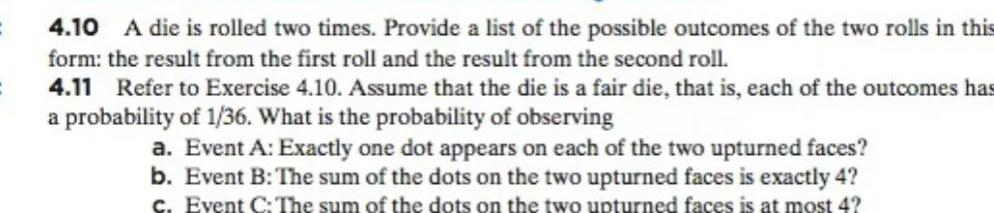Question:

# 4 10 A die is rolled two times Provide a list of the

Last updated: 9/19/20234 10 A die is rolled two times Provide a list of the possible outcomes of the two rolls in this form the result from the first roll and the result from the second roll 4 11 Refer to Exercise 4 10 Assume that the die is a fair die that is each of the outcomes has a probability of 1 36 What is the probability of observing a Event A Exactly one dot appears on each of the two upturned faces b Event B The sum of the dots on the two upturned faces is exactly 4 c Event C The sum of the dots on the two upturned faces is at most 4